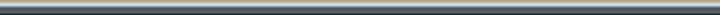HOME >> Tutorials >> Conditionals# ConditionsThis tutorial will teach you about conditions on the TI-83+.

Condtions are used in a program to determine if a certain block of code you set will be excecuted or not. For example, in a game like Tron, where you are a continous snake and must avoid walls and trap your enemy, the program must be able to tell if you run into a wall. So IF you run into a wall, THEN end the program (as it would be a game over).

To make a condition inside a program, you would do this:

(earlier code in the program)
:Then
:(do this)
:(do this)
:End
(remainder of program)

In this example, "your condition is true", can be any condition. For example, if you put "A=5" in this section, then when the program reached "If A=5", it would look at the value stored in the variable "A". If this value equaled 5, then the program would continue and execute the commands after the If statement and ignore the "End". If the value in A was not 5, the program will SKIP all commands after the If statement and will not continue excecuting commands again until it reaches its corrsponding "End"

The command "Then" must be used in all conditions were the number of commands to be executed if the statement is true is greater than 1. Should you ONLY need one command executed if and only if the statement is true, than you could do this:

(earlier part of program)
:(do this)
(remainder of program)

As mentioned earlier, if you do not have a "Then", (in which case you also don't need an "End", it is implied that only the one immediate command following the If statement should be excecuted should the condition be true.

Let's make a program using this knowledge that will count to ten and stop. For the sake of this tutorial, I will make comments after a "//" which should NOT be entered into a program. This will not work inside a program. Create a new program and enter:

:0->A
:Lbl 00 //Creates a label in the program, will be ignored for now
:A+1->A
:Disp A //Displays the value inside the variable A
:If A=10
:Stop //This command will stop the program
:Goto 00 //This statement will goto the place in the program where the "Lbl" with the corresponding two ending digits is located (In this case it is "00")

There are much better ways to create this program, but it would require a lot of explaining that I will do in another tutorial and it may confuse you to learn too much all at one time. (I honestly don't mean any offense by that).

Run the program. Look at the program. Does it make sense? The program will keep going through itself and going back to "Lbl 00". Each time it goes through, A is incremented by one. When A reaches 10, the program will stop.

You now know the basics of conditions

Now, we will take a closer look at conditions and find out what makes them work and some advanced meathods of creating conditions.

If statements use what is called "Boolean Logic". In that everything is either Off or On. 1 represents On, and 0 represents Off. "Not(" is a command that will take the opposite of a number using Boolean Logic. Also, any nonzero number is considered On, not just 1. See for yourself. On the homescreen type:

not(5) //press enter to get "0"
not(Ans) //press enter to get "1"

The opposite of 5, since it is non-zero, is zero. The opposite of 0, in every case, will then be 1.

If statements use this to determine if a statemnet is true or not. Say you have If A=5, same as last time, the program will check the statement to see if it is true. If it is, a 1 is assigned to the condition. If it is not true, a 0 is assigned to the condition. Now the statement has only two possible answers 1 or 0. The If statement will consider itself true if the assigned value is nonzero. This type of thing does not nessisarily need to be in an If statement. Have you ever seen anything like this?:

:X+(A=26)-(A=24)->X

This, at first can look very confusing. What could A be? Say A equals 24. The conditions inside the parentheses will be anylized. (A=26) will become a zero as this is false, and (A=24) will become a one as it is true. What you end up with is "X+0-1->X" or "X-1->X" if A=26, then going back through this line, we would end up with "X+1->X". However, only when A=24 or A=26, will the value inside X ever be changed as all you would get in any other condition is "X+0-0->X" or "X->X".

There are times that an adaption off of the above command may come in very useful. Conditions like this can be inserted into almost anything. Use your imagination. "There is a better way to do it. FIND it." - Anonymous